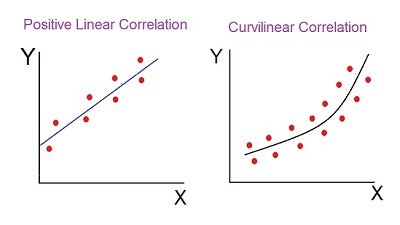Skip to content

# Methods of Studying Correlation

The Correlation is a statistical tool used to measure the relationship between two or more variables, i.e. the degree to which the variables are associated with each other, such that the change in one is accompanied by the change in another.

The correlation is said to be linear when the change in the amount of one variable tends to bear a constant ratio to the amount of change in another variable. Whereas, the non-linear or curvilinear correlation is when the ratio of the amount of change in one variable to the amount of change in another variable is not constant.These figures clearly show the difference between the linear and non-linear correlation. To determine the linearity and non-linearity among the variables and the extent to which these are correlated, following are the important methods used to ascertain these:

1. Scatter Diagram Method
2. Karl Pearson’s Coefficient of Correlation
3. Spearman’s Rank Correlation Coefficient; and
4. Methods of Least Squares

Among these, the first method, i.e. scatter diagram method is based on the study of graphs while the rest is mathematical methods that use formulae to calculate the degree of correlation between the variables.  The researcher may apply either of these methods on the basis of the nature of variables being considered in ascertaining the association between them.

### 1 Comment »

error: Content is protected !!